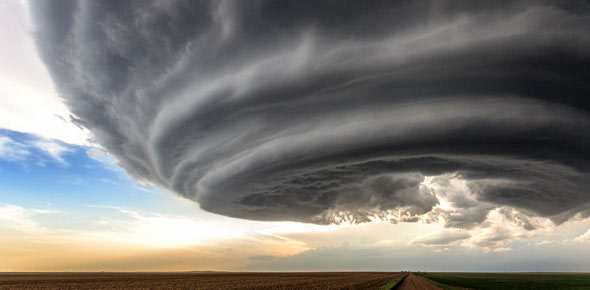# Physical Science Tutor Competency Quiz

10 Questions | Attempts: 396Settings• 1.
An alternating-current-carrying wire induces a current in another nearby wire. This phenomenon is the basis of operation of which of the following devices?
• A.

Motor

• B.

Generator

• C.

Galvanometer

• D.

Transformer

• 2.
The buoyant force exerted by a fluid on an entirely submerged object is equal to _________________.
• A.

The mass of the submerged object

• B.

The gravitational force acting on the submerged object

• C.

The density of the submerged object

• D.

The weight of the fluid displaced by the submerged object

• 3.
What is the kinetic energy of a 12-kg toy car moving at a velocity of 3m/s?
• A.

36J

• B.

54J

• C.

108J

• D.

18J

• 4.
Compounds that have the same molecular formula but different chemical structures are called _________________.
• A.

Covalent

• B.

Ionic

• C.

Metallic

• D.

Isomers

• 5.
___________ states that the volume of a given amount of gas held at constant temperature varies inversely with the applied pressure when the temperature and mass are constant.
• A.

Boyle's Law

• B.

Charles' Law

• C.

• D.

Ideal Gas Law

• 6.
A bond in which one or more electrons from one atom are removed and attached to another atom, resulting in positive and negative ions which attract each other, is called a __________.
• A.

Covalent bond

• B.

Ionic bond

• C.

Metallic bond

• D.

Hydrogen bond

• 7.
When dissolved in water, bases have all of the following properties except _______________.
• A.

Have a sour taste.

• B.

Change red litmus to blue

• C.

Have a slippery feeling

• D.

Conduct electricity

• 8.
The reaction of methane with oxygen has the following unbalanced equation: CH₄(g) + O₂(g) CO₂(g) + H₂O(l). The coefficient for oxygen in the balanced equation is ______.
• A.

1

• B.

2

• C.

3

• D.

5

• 9.
What wave propagation principle accounts for the apparent increase in frequency as a train whistle approaches and the apparent decrease in frequency as it moves away?
• A.

Refraction

• B.

Reflection

• C.

Diffraction

• D.

Doppler effect

• 10.
Melting ice is an example of increasing ___________.
• A.

Entropy

• B.

Enthalpy

• C.

Heat loss

• D.

Gibbs free energy

## Related TopicsBack to top
×

Wait!
Here's an interesting quiz for you.